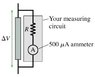# Ammeter Voltmeter problem

• rayhan619

## Homework Statement

A circuit you're building needs a voltmeter that goes from 0 to a full-scale reading of 5.0 V. Unfortunately, the only meter in the storeroom is an ammeter that goes from 0 to a full-scale reading of 500 microA. It is possible to use this meter to measure voltages by putting in a measuring circuit as shown in the figure. (Figure attached)
What value of R must you use so that the meter will go to full scale when the potential difference Delta V is 5.0 V? Assume that the ammeter is ideal.

I = VR

## The Attempt at a Solution

#### Attachments

•1.jpg
8.2 KB · Views: 1,311

When you connect the ammeter with a high resistance R in series across a measuring circuit, the current in the ammeter should be 500 mA. Delta V is given, Find R.

Delta V = IR
I = 500 microA = 500*10^-6 A
Delta V = 5 V
R = V/I = 5 V/(500*10^-6 A) = 1*10^4 Ohm

Is this correct?

Yes.

I appreciate it.